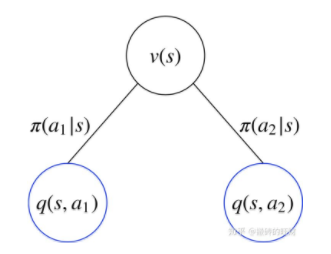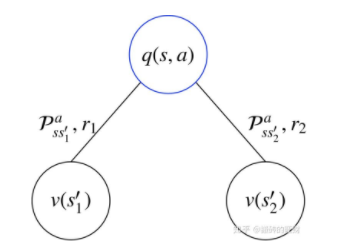• ## 马尔可夫

千次阅读 2011-05-12 14:40:00
<br />马尔可夫性质是概率论中的一个概念。当一个随机过程在给定现在状态及所有过去状态情况下，其未来状态的条件概率分布仅依赖于当前状态；换句话说，在给定现在状态时，它与过去状态（即该过程的历史路径）...

马尔可夫性质是概率论中的一个概念。当一个随机过程在给定现在状态及所有过去状态情况下，其未来状态的条件概率分布仅依赖于当前状态；换句话说，在给定现在状态时，它与过去状态（即该过程的历史路径）是条件独立的，那么此随机过程即具有马尔可夫性质。具有马尔可夫性质的过程通常称之为马尔可夫过程。
数学上，如果X(t),t > 0为一个随机过程，则马尔可夫性质就是指$/mathrm{Pr}/big[X(t+h) = y /,|/, X(s) = x(s), s /leq t/big] = /mathrm{Pr}/big[X(t+h) = y /,|/, X(t) = x(t)/big], /quad /forall h > 0.$

马尔可夫过程通常称其为（时间）齐次，如果满足$/mathrm{Pr}/big[X(t+h) = y /,|/, X(t) = x(t)/big] = /mathrm{Pr}/big[X(h) = y /,|/, X(0) = x(0)/big], /quad /forall t, h > 0,$

除此之外则被称为是（时间）非齐次的。齐次马尔可夫过程通常比非齐次的来得简单，构成了最重要的一类马尔可夫过程。
某些情况下，明显的非马尔可夫过程也可以通过扩展“现在”和“未来”状态的概念来构造一个马尔可夫表示。设X为一个非马尔可夫过程。我们就可以定义一个新的过程Y，使得每一个Y的状态表示X的一个时间区间上的状态，用数学方法来表示，即，$Y(t) = /big/{ X(s) : s /in [a(t), b(t)] /, /big/}.$

如果Y具有马尔可夫性质，则它就是X的一个马尔可夫表示。 在这个情况下，X也可以被称为是二阶马尔可夫过程。更高阶马尔可夫过程也可类似地来定义。
具有马尔可夫表示的非马尔可夫过程的例子，例如有移动平均时间序列。
最有名的马尔可夫过程为马尔可夫链，但不少其他的过程，包括布朗运动也是马尔可夫过程。

隐马尔可夫模型（Hidden Markov Model，HMM）是统计模型，它用来描述一个含有隐含未知参数的马尔可夫过程。其难点是从可观察的参数中确定该过程的隐含参数。然后利用这些参数来作进一步的分析，例如模式识别。
在正常的马尔可夫模型中，状态对于观察者来说是直接可见的。这样状态的转换概率便是全部的参数。而在隐马尔可夫模型中,状态并不是直接可见的，但受状态影响的某些变量则是可见的。每一个状态在可能输出的符号上都有一概率分布。因此输出符号的序列能够透露出状态序列的一些信息。

目录
[隐藏]

1 马尔可夫模型的演化2 使用隐马尔可夫模型
2.1 具体实例2.2 隐马尔可夫模型的应用 3 历史4 参见5 注解6 参考书目7 外部连接
[编辑]马尔可夫模型的演化
上边的图示强调了HMM的状态变迁。有时，明确的表示出模型的演化也是有用的,我们用x（t1） 与x（t2）来表达不同时刻t1 和t2的状态。在这个图中,每一个时间块（x(t), y(t)）都可以向前或向后延伸。通常，时间的起点被设置为t=0 或 t=1.
[编辑]使用隐马尔可夫模型
HMM有三个典型(canonical)问题:
已知模型参数，计算某一特定输出序列的概率.通常使用forward算法解决.已知模型参数，寻找最可能的能产生某一特定输出序列的隐含状态的序列.通常使用Viterbi算法解决.已知输出序列，寻找最可能的状态转移以及输出概率.通常使用Baum-Welch算法以及Reversed Viterbi算法解决.
另外,最近的一些方法使用Junction tree算法来解决这三个问题。
[编辑]具体实例
假设你有一个住得很远的朋友,他每天跟你打电话告诉你他那天做了什么.你的朋友仅仅对三种活动感兴趣:公园散步,购物以及清理房间.他选择做什么事情只凭天气.你对于他所住的地方的天气情况并不了解,但是你知道总的趋势.在他告诉你每天所做的事情基础上,你想要猜测他所在地的天气情况.
你认为天气的运行就像一个马尔可夫链.其有两个状态 "雨"和"晴",但是你无法直接观察它们,也就是说,它们对于你是隐藏的.每天,你的朋友有一定的概率进行下列活动:"散步", "购物", 或 "清理". 因为你朋友告诉你他的活动,所以这些活动就是你的观察数据.这整个系统就是一个隐马尔可夫模型HMM.
你知道这个地区的总的天气趋势,并且平时知道你朋友会做的事情.也就是说这个隐马尔可夫模型的参数是已知的.你可以用程序语言(Python)写下来:
states = ('Rainy', 'Sunny')

observations = ('walk', 'shop', 'clean')

start_probability = {'Rainy': 0.6, 'Sunny': 0.4}

transition_probability = {
'Rainy' : {'Rainy': 0.7, 'Sunny': 0.3},
'Sunny' : {'Rainy': 0.4, 'Sunny': 0.6},
}

emission_probability = {
'Rainy' : {'walk': 0.1, 'shop': 0.4, 'clean': 0.5},
'Sunny' : {'walk': 0.6, 'shop': 0.3, 'clean': 0.1},
}

在这些代码中,start_probability代表了你对于你朋友第一次给你打电话时的天气情况的不确定性(你知道的只是那个地方平均起来下雨多些).在这里,这个特定的概率分布并非平衡的,平衡概率应该接近（在给定变迁概率的情况下）{'Rainy': 0.571, 'Sunny': 0.429}< transition_probability 表示基于马尔可夫链模型的天气变迁,在这个例子中,如果今天下雨,那么明天天晴的概率只有30%.代码emission_probability 表示了你朋友每天做某件事的概率.如果下雨,有 50% 的概率他在清理房间;如果天晴,则有60%的概率他在外头散步.
这个例子在Viterbi算法页上有更多的解释。
[编辑]隐马尔可夫模型的应用
语音识别、中文断词/分词或光学字符识别机器翻译生物信息学 和 基因组学
基因组序列中蛋白质编码区域的预测对于相互关联的DNA或蛋白质族的建模从基本结构中预测第二结构元素 还有更多...

马尔可夫链，因俄罗斯数学家安德烈·马尔可夫得名，是数学中具有马尔可夫性质的离散时间随机过程。该过程中，在给定当前知识或信息的情况下，只有当前的状态用来预测将来，过去（即当前以前的历史状态）对于预测将来（即当前以后的未来状态）是无关的。
在马尔可夫链的每一步，系统根据概率分布，可以从一个状态变到另一个状态,也可以保持当前状态。状态的改变叫做过渡，与不同的状态改变相关的概率叫做过渡概率。随机漫步就是马尔可夫链的例子。随机漫步中每一步的状态是在图形中的点，每一步可以移动到任何一个相邻的点，在这里移动到每一个点的概率都是相同的(无论之前漫步路径是如何的)。

目录
[隐藏]

1 历史2 定义3 性质
3.1 可还原性3.2 周期性3.3 重现性3.4 各态历遍性3.5 律动性3.6 平稳状态分析和极限分布 4 可反转马尔可夫链5 有限状态空间中的马尔可夫链6 科学应用
6.1 统计6.2 生物6.3 地理6.4 因特网应用6.5 马尔可夫模仿文本生成器 7 参看8 参考文献9 外部链接
历史
马尔可夫在1906年首先做出了这类过程。而将此一般化到可数无限状态空间是由柯尔莫果洛夫在1936年给出的。马尔可夫链与布朗运动以及遍历假说这两个二十世纪初期物理学重要课题是相联系的，但马尔可夫寻求的似乎不仅于数学动机，名义上是对于纵属事件大数法则的扩张。
定义
马尔可夫链是随机变量X1,X2,X3...的一个数列。这些变量的范围，即他们所有可能取值的集合，被称为“状态空间”，而Xn的值则是在时间n的状态。如果Xn + 1对于过去状态的条件概率分布仅是Xn的一个函数，则$P(X_{n+1}=x|X_0, X_1, X_2, /ldots, X_n) = P(X_{n+1}=x|X_n). /,$

这里x为过程中的某个状态。上面这个恒等式可以被看作是马尔可夫性质。
性质
可还原性
马尔可夫链是由一个条件分布来表示的$P(X_{n+1}| X_n)/,$

这被称为是随机过程中的“转移概率”。这有时也被称作是“一步转移概率”。二、三，以及更多步的转移概率可以导自一步转移概率和马尔可夫性质：$P(X_{n+2}|X_n) = /int P(X_{n+2},X_{n+1}|X_n)/,dX_{n+1} = /int P(X_{n+2}|X_{n+1}) /, P(X_{n+1}|X_n) /, dX_{n+1}$

同样，$P(X_{n+3}|X_n) = /int P(X_{n+3}|X_{n+2}) /int P(X_{n+2}|X_{n+1}) /, P(X_{n+1}|X_n) /, dX_{n+1} /, dX_{n+2}$

这些式子可以通过乘以转移概率并求k − 1次积分来一般化到任意的将来时间n + k。
周期性
边际分布 P(Xn)是在时间为n时的状态的分布。初始分布为P(X0)。该过程的变化可以用以下的一个时间步幅来描述：$P(X_{n+1}) = /int P(X_{n+1}|X_n)/,P(X_n)/,dX_n$

这是Frobenius-Perron equation的一个版本。这时可能存在一个或多个状态分布π满足$/pi(X) = /int P(X|Y)/,/pi(Y)/,dY$

平稳分布是否存在，以及如果存在是否唯一，这是由过程的特定性质决定的。“不可约”是指每一个状态都可来自任意的其它状态。当存在至少一个状态经过一个固定的时间段后连续返回，则这个过程被称为是“周期的”。
重现性
各态历遍性
律动性
平稳状态分析和极限分布
可反转马尔可夫链
可反转马尔可夫链类似于应用贝叶斯定理来反转一个条件概率:/begin{align} /Pr(X_{n}=i/mid X_{n+1}=j) & = /frac{/Pr(X_n = i, X_{n+1} = j)}{/Pr(X_{n+1} = j)} // & = /frac{/Pr(X_{n} = i)/Pr(X_{n+1} = j/mid X_n=i)}{/Pr(X_{n+1} = j)}. /end{align}

以上就是反转的马尔可夫链。因而，如果存在一个π，使得:$/pi_i p_{ij} = /pi_j p_{ji}./,$

那么这个马尔可夫链就是可反转的。
这个条件也被称为细致平衡 (detailed balance)条件。
对于所有的i求和:$/sum_i /pi_i p_{ij} = /pi_j/,$

所以，对于可反转马尔可夫链，π总是一个平稳分布。
有限状态空间中的马尔可夫链
如果状态空间是有限的，则转移概率分布可以表示为一个具有(i,j)元素的矩阵，称之为“转移矩阵”：$P_{ij} = P(X_{n+1}=j/mid X_n=i) /,$

对于一个离散状态空间，k步转移概率的积分即为求和，可以对转移矩阵求k次幂来求得。就是说，如果$/mathbf{P}$是一步转移矩阵，$/mathbf{P}^k$就是k步转移后的转移矩阵。
平稳分布是一个满足以下方程的矢量$/mathbf{P}/pi^* = /pi^*$.

在此情况下，稳态分布π * 是一个对应于特征根为1的、该转移矩阵的特征矢量。
如果转移矩阵$/mathbf{P}$不可约，并且是非周期的，则$/mathbf{P}^k$收敛到一个每一列都是不同的平稳分布 π * ，并且，$/lim_{k/rightarrow/infty}/mathbf{P}^k/pi=/pi^*$,

独立于初始分布π。这是由Perron-Frobenius theorem所指出的。
正的转移矩阵（即矩阵的每一个元素都是正的）是不可约和非周期的。矩阵被称为是一个随机矩阵，当且仅当这是某个马尔可夫链中转移概率的矩阵。
注意：在上面的定式化中，元素(i,j)是由j转移到i的概率。有时候一个由元素(i,j)给出的等价的定式化等于由i转移到j的概率。在此情况下，转移矩阵仅是这里所给出的转移矩阵的转置。另外，一个系统的平稳分布是由该转移矩阵的左特征矢量给出的，而不是右特征矢量。
转移概率独立于过去的特殊况为熟知的Bernoulli scheme。仅有两个可能状态的Bernoulli scheme被熟知为伯努利过程
科学应用
统计
马尔可夫链通常用来建模排队理论和统计学中的建模，还可作为信号模型用于熵编码技术，如算术编码（著名的LZMA数据压缩算法就使用了马尔可夫链与类似于算术编码的区间编码）。
生物
马尔可夫链也有众多的生物学应用，特别是人口过程，可以帮助模拟生物人口过程的建模。隐蔽马尔可夫模型还被用于生物信息学，用以编码区域或基因预测。
地理
马尔可夫链最近的应用是在地理统计学（geostatistics）中。其中，马尔可夫链用在基于观察数据的二到三维离散变量的随机模拟。这一应用类似于“克里金”地理统计学（Kriging geostatistics），被称为是“马尔可夫链地理统计学”。这一马尔可夫链地理统计学方法仍在发展过程中。
因特网应用
谷歌所使用的网页排序算法（PageRank）就是由马尔可夫链定义的。马尔可夫模型也被应用于分析用户浏览网页的行为。一阶或者二阶的马尔可夫模型可以用于对一个用户从某一网络链接转移到另一链接的行为进行建模，然后这些模型可以用于对用户之后的浏览行为进行预测。
马尔可夫模仿文本生成器
马尔可夫过程，能为给定样品文本，生成粗略，但看似真实的文本：他们被用于众多供消遣的“模仿生成器”软件。马尔可夫链还被用于谱曲。

展开全文transition 算法 scheme
• 马尔可夫马尔可夫马尔可夫马尔可夫马尔可夫马尔可夫预测 马尔可夫
•研究论文
• 详解介绍马尔可夫链，里面包含一系列简单实例案例，容易理解
• 介绍了马尔可夫模型一类重要的随机过程，它的原始模型马尔可夫链，由俄国数学家Α.Α.马尔可夫于1907年提出。人们在实际中常遇到具有下述特性的随机过程：在已知它所处的状态的条件下，它未来的演变不依赖于它以往的...
• 图像显著性--马尔可夫--Saliency Detection via Absorbing Markov Chain
• MATLAB编写的语音识别程序-基于隐马尔可夫模型的语音识别
• 通过对马尔可夫模型进行深入的分析的基础上对隐马尔科夫模型做了详细的讨论，对马尔科夫模型在语音识别、疾病分析等方面的应用做了介绍，同时针对隐马尔科夫模型在估值问题、解码问题和学习问题等经典问题上的应用做...
• 若上述网络是无向的，则是无向图模型，又称 马尔可夫随机场或者马尔可夫网络 。 如果在给定某些条件的前提下，研究这个马尔可夫随机场，则得到 条件随机场 。 如果使用条件随机场解决标注问题，并且进一步将...
• ## 马尔可夫过程

千次阅读 2019-10-14 20:23:34
马尔可夫过程 强化学习基于马尔可夫过程，研究的问题都可以抽象成马尔可夫过程。其定义为满足马尔可夫性质的随机过程。 马尔可夫性质：通俗来讲，即当前状态包含了所有相关的历史，只要当前的状态已知，下一个状态...
马尔可夫过程
强化学习基于马尔可夫过程，研究的问题都可以抽象成马尔可夫过程。其定义为满足马尔可夫性质的随机过程。 马尔可夫性质：通俗来讲，即当前状态包含了所有相关的历史，只要当前的状态已知，下一个状态的发生可能性就已经确定，不需要知道从开始到当前状态所经历的具体的状态变换。

P

(

s

t

+

1

∣

s

t

)

=

P

(

s

t

+

1

∣

s

t

,

s

t

−

1

,

s

t

−

2

.

.

.

s

0

)

P(s_{t+1}|s_t)=P(s_{t+1}|s_t,s_{t-1},s_{t-2}...s_0)

马尔可夫过程奖励
马尔可夫过程可以用一个元组

(

S

,

A

,

P

,

R

,

γ

)

(S,A,P,R,γ)

,

S

S

为状态空间集合，

A

A

为动作空间集，

P

P

为状态转移概率分布矩阵，

R

R

为各个状态

s

s

的奖励集，

γ

γ

折扣因（0-1） 奖励（回报） 每一个状态

s

s

都有一个奖励值，集合为

R

R

，在

t

t

时刻从状态

s

t

s_t

开始，到这个回合（episode）结束，所能获得的奖励总和称为回报。

G

t

=

r

t

+

1

+

γ

r

t

+

2

+

γ

2

r

t

+

3

+

γ

3

r

t

+

4

+

γ

4

r

t

+

5

+

.

.

.

G_t=r_{t+1}+γr_{t+2}+γ^2r_{t+3}+γ^3r_{t+4}+γ^4r_{t+5}+...

G

t

=

r

t

+

1

+

γ

G

t

+

1

G_t=r_{t+1}+γG_{t+1}

其中

γ

γ

为折扣因子，取值0-1，其代表的意义为，当前眼前的奖励值与未来奖励值的占比关系。从现实生活考虑，未来的回报不确定性因素高，需要打个折扣。当

γ

γ

趋于1时，表示对未来的回报越看重，更加考虑未来的收益，不局限于眼前的利益。相反，趋于0时，表示注重眼前的收益。 由于每个episode所经历的状态过程是随机的，不确定性高，但是我们需要一个稳定的奖励值来指导Agent,因此我们可以对回报函数求期望，就得到一个价值函数

V

(

s

)

V(s)

。 策略 策略

π

π

是指智能体在某个时刻的状态，根据策略决策应该选择哪个动作。 确定性策略：

s

s

->

a

a

的映射，即在状态

s

s

，选择动作

a

a

的概率为1. 随机策略：

π

(

a

∣

s

)

π(a|s)

，表示在状态

s

s

下，选择动作

a

a

的概率值，该值不一定为1，即面对状态

s

s

，agent依概率选择动作，则有可能会选到概率值小的动作（可能性较小而已）。
价值函数
状态的奖励

R

R

是状态的立即回报，是‘眼前的利益’ 状态价值函数 在某一个策略

π

π

下，状态

s

t

s_t

的价值函数，即为根据这个策略，一步步走到结束，每个状态的奖励的加和期望。

V

π

（

s

t

）

=

E

π

[

G

t

∣

s

t

]

=

E

π

(

r

t

+

1

+

γ

G

s

t

+

1

)

V_π（s_t）=E_π[G_t|s_t]=E_π(r_{t+1}+γG_{s_{t+1}})

=

E

π

(

r

t

+

1

)

+

E

π

(

γ

G

s

t

+

1

)

=

R

t

+

1

+

γ

V

π

(

s

t

+

1

)

=E_π(r_{t+1})+E_π(γG_{st+1})=R_{t+1}+γV_π(s_{t+1})

当前状态价值，等于该状态的立即回报的期望(下一个状态是不确定，因此不能确定立即回报，要取期望)+下个状态打了折扣的价值，状态价值函数描述了该状态的好坏。 动作价值函数 在某一个策略

π

π

下，状态

s

t

s_t

，选择具体某个动作

a

t

a_t

的价值函数，即选择完动作后，一直走到结束的所有奖励加和的期望。

Q

π

(

s

t

,

a

t

)

=

E

π

[

G

t

∣

s

t

,

a

t

]

Q_π(s_t,a_t)=E_π[G_t|s_t,a_t]

动作价值函数描述了在状态

s

t

s_t

下，选择该动作的好坏。
状态价值函数和动作价值函数的关系要求某个状态

s

s

的状态价值函数，主要考虑，从这个状态出发，一共有几个动作可以选，每个动作对应的动作价值函数的累加和就是该状态的价值。

V

π

(

s

)

=

∑

π

(

a

i

∣

s

)

q

π

(

s

,

a

i

)

V_π(s)=\sum π(a_i|s)q_π(s,a_i)

对于确定性策略，对于某个状态，选择的动作是确定的，概率是1，因此

π

(

a

∣

s

)

=

1

π(a|s)=1

,上式就可以写成，

V

π

(

s

)

=

q

π

(

s

,

a

)

V_π(s)=q_π(s,a)

，状态价值函数就等于了动作价值函数。要求某一状态下

s

s

下，确定选择某个动作

a

a

后，这个动作的价值函数，可以考虑，动作确定后，他就会转变成下一个状态，获得一个立即回报，但是转变成哪个状态是不确定的，和状态转移概率有关，因此当前动作的价值等于可能转变成的下一个状态的

s

′

s\prime

的状态价值累计和以及状态改变带来的立即回报。

q

π

(

s

,

a

)

=

∑

P

s

s

′

a

(

r

i

+

v

π

(

s

′

i

)

)

=

R

s

a

+

∑

P

s

s

′

a

v

π

(

s

′

i

)

q_π(s,a)=\sum P_{ss\prime}^a (r_i+v_π(s\prime_i))=R_s^a+\sum P_{ss\prime}^a v_π(s\prime_i)

将

q

π

(

s

,

a

)

q_π(s,a)

带入

V

π

(

s

)

V_π(s)

可以得到：

V

π

(

s

)

=

∑

π

(

a

i

∣

s

)

[

R

s

a

+

∑

P

s

s

′

a

v

π

(

s

′

i

)

]

V_π(s)=\sum π(a_i|s)[R_s^a+\sum P_{ss\prime}^a v_π(s\prime_i)]

该方程就是贝尔曼方程(Bellman)。 用矩阵形式表示：

V

π

⃗

=

R

s

a

⃗

+

γ

P

s

s

′

V

π

⃗

\vec{V_π}=\vec{R_s^a}+γP_{ss\prime}\vec{V_π}

和

V

π

(

s

t

)

=

R

t

+

1

+

γ

V

π

(

s

t

+

1

)

V_π(s_t)=R_{t+1}+γV_π(s_{t+1})

本质上是一致的，当前状态的价值=立即回报+下一个状态的价值 同理可以推出

q

π

(

s

,

a

)

=

R

s

a

+

∑

P

s

s

′

a

∑

π

(

a

i

∣

s

′

)

q

π

(

s

′

,

a

i

)

q_π(s,a)=R_s^a+\sum P_{ss\prime}^a \sum π(a_i|s\prime)q_π(s\prime,a_i)

通过贝尔曼方程，当状态转移矩阵和策略已知的条件下，就可以通过迭代求出最优策略。
最优策略
对于MDP问题，一定存在一个最优策略

π

∗

π^*

,设该策略下的状态价值函数为

V

∗

(

s

)

V^*(s)

,动作价值函数

Q

∗

(

s

,

a

)

Q^*(s,a)

,则有下式成立

Q

∗

(

s

,

a

)

≥

Q

π

(

s

,

a

)

,

V

∗

(

s

)

≥

V

(

s

)

对

任

意

的

策

略

π

Q^*(s,a)\geq Q_π(s,a),V^*(s) \geq V(s) 对任意的策略π

定理：
对于任何MDP，存在一个最优策略，比任何其他策略更好，或至少相等；所有的最优策略有相同的最优价值函数；所有的最优策略有相同的动作价值函数；
因此，求最优策略可以通过求最优价值函数，转换为求价值函数的最大值问题。
动态规划
动态规划是需要在状态转移概率矩阵和回报函数已知的情况下，可以通过迭代求解得到最优策略。 动态规划问题两个性质： 最优子结构：保证能够使用最优性原理(多阶段决策过程的最优决策序列具有这样的性质：不论初始状态和初始决策如何，对于前面决策所造成的某一状态而言，其后各阶段的决策序列必须构成最优策略),问题可以转化成求子问题最优。 子问题重叠：子问题反复出现，可以缓存子问题和重利用子问题的解。
策略迭代
给定初始策略

π

k

π_k

，计算出该策略下的状态

V

π

k

V_{π_k}

和动作价值函数

V

π

k

V_{π_k}

，根据贪心策略

π

k

+

1

(

s

,

a

)

=

m

a

x

a

Q

π

k

(

s

,

a

)

π_{k+1}(s,a)=max_aQ_{π_k}(s,a)

，得到新的策略

π

k

+

1

π_{k+1}

,然后在新的策略下，求出新的价值函数，反复迭代，直到价值函数和策略收敛，就求出了最优策略。 策略评估：

V

π

k

+

1

(

s

)

=

∑

π

(

a

i

∣

s

)

[

R

s

a

+

γ

∑

P

s

s

′

a

V

π

k

(

s

′

i

)

]

V_{πk+1}(s)=\sum π(a_i|s)[R_s^a+γ\sum P_{ss\prime}^a V_{πk}(s\prime_i)]

即求出该策略下价值函数最终的收敛值，贝尔曼方程中，求解当前状态

s

s

下的价值

V

k

+

1

(

s

)

V_{k+1}(s)

等于立即回报+下一个状态

s

′

s\prime

的价值

V

k

+

1

(

s

′

)

V_{k+1}(s\prime)

，但是我们不知道下一个状态

s

′

s\prime

的价值

V

k

+

1

(

s

′

)

V_{k+1}(s\prime)

是多少，因此这里采用的是上一次迭代的

s

′

s\prime

的价值

V

k

(

s

′

)

V_{k}(s\prime)

替代。因此在一轮迭代中，可以对将所有的状态的价值函数进行更新，然后进行下一轮，直到价值收敛了，就求出了该策略下的状态价值函数。 策略改进： 对于小问题，第一轮状态价值函数更新收敛，求出某策略下的状态价值函数后，根据贪心策略，可能就能得到最终最优策略。而复杂大问题，此时根据贪心策略得到新的策略，该策略还不是最优策略，需要重新进行策略评估，然后在进行策略改进，不断进行迭代，直到策略收敛。
价值迭代
策略迭代中，需要先进行策略评估，先求出当前策略下的价值函数，在根据价值函数进行策略改进。如果将贝尔曼方程：

V

(

s

)

=

∑

π

(

a

i

∣

s

)

[

R

s

a

+

γ

∑

P

s

s

′

a

V

(

s

′

i

)

]

V(s)=\sum π(a_i|s)[R_s^a+γ\sum P_{ss\prime}^a V(s\prime_i)]

改成

V

k

+

1

(

s

)

=

m

a

x

a

[

R

s

a

+

γ

∑

P

s

s

′

a

V

k

(

s

′

i

)

]

V_{k+1}(s)=max_a[R_s^a+γ\sum P_{ss\prime}^a V_k(s\prime_i)]

价值迭代不涉及策略，（但是需要知道具体状态下，下一个所有的状态以及相应的转换概率）直接通过价值函数迭代逼近最优价值函数，跳过了策略改进的步骤。实际上改进过程已经通过

m

a

x

a

max_a

实现了。贝尔曼方程是求的期望，改进后的价值方程是贝尔曼优化方程。 同理，动作价值函数也有迭代公式：

Q

π

+

1

(

s

,

a

)

=

R

s

a

+

γ

∑

P

s

s

′

a

m

a

x

a

Q

π

(

s

′

,

a

′

)

Q_{π+1}(s,a)=R_s^a+γ\sum P_{ss\prime}^amax_aQ_π(s\prime,a\prime)

广义策略迭代
在策略迭代中，策略改进，必须在策略评估迭代收敛后再进行。然而，实际上并不需要策略评估收敛后再进行策略改进，值迭代就是最极端的例子，不进行评估就进行策略改进(实际暗中是进行了策略评估的一次更新)，同样也可以得到最优策略。因此还有很多介于两者之间的方法，只要所有的状态价值和策略在不断地更新迭代，则最终一定会收敛到最优价值函数和最优策略，称为广义策略迭代。即只强调价值函数和策略在不断迭代提升，不关注具体的细节。强化学习都可以很好地描述成广义策略迭代。
展开全文动态规划 强化学习
• MCMC马尔可夫链蒙特卡洛法入门教程，内含code
• 马尔可夫过程 马尔科度模型 马尔可夫链 关键参数 1.1 马尔可夫过程 马尔可夫过程（Markov process）是一类随机过程。由俄国数学家A.A.马尔可夫于1907年提出。该过程具有如下特性：在已知目前状态（现在）的条件下...
马尔可夫过程马尔科度模型马尔可夫链关键参数
1.1 马尔可夫过程
   马尔可夫过程（Markov process）是一类随机过程。由俄国数学家A.A.马尔可夫于1907年提出。该过程具有如下特性：在已知目前状态（现在）的条件下，它未来的演变（将来）不依赖于它以往的演变 (过去 )。

1.2 马尔可夫模型
一个马尔科夫过程就是指过程中的每个状态的转移只依赖于之前的 n个状态，这个过程被称为 n阶马尔科夫模型，其中 n是影响转移状态的数目。最简单的马尔科夫过程就是一阶过程，每一个状态的转移只依赖于其之前的那一个状态，这也是后面很多模型的讨论基础，很多时候马尔科夫链、隐马尔可夫模型都是只讨论一阶模型，甚至很多文章就将一阶模型称之为马尔科夫模型，现在我们知道一阶只是一种特例而已了。
对于一阶马尔科夫模型，如果第 i 时刻上的取值依赖于且仅依赖于第 i−1 时刻的取值，即​ 从这个式子可以看出，xi 仅仅与 xi-1有关，二跟他前面的都没有关系了，这就是一阶过程。
1.3 马尔可夫链
​ 时间和状态都是离散的马尔可夫过程称为马尔可夫链,简记为Xn=X(n),n=0,1,2…马尔可夫链是随机变量X1,X2,X3…的一个数列。
总结：马尔科夫过程指的是一个状态不断演变的过程，对其进行建模后称之为马尔科夫模型，在一定程度上，马尔科夫过程和马尔科夫链可以打等号的。
1.4 关键参数
（1）关键概念——状态空间
马尔可夫链是随机变量X1,X2,X3…Xn所组成的一个数列，每一个变量Xi 都有几种不同的可能取值，即他们所有可能取值的集合，被称为“状态空间”，而Xn的值则是在时间n的状态。
（2）关键概念——转移概率(Transition Probability）
马尔可夫链可以用条件概率模型来描述。我们把在前一时刻某取值下当前时刻取值的条件概率称作转移概率。上面是一个条件概率，表示在前一个状态为s的条件下，当前状态为t的概率是多少。
（3）关键概念——转移概率矩阵
很明显，由于在每一个不同的时刻状态不止一种，所以由前一个时刻的状态转移到当前的某一个状态有几种情况，那么所有的条件概率会组成一个矩阵，这个矩阵就称之为“转移概率矩阵”。比如每一个时刻的状态有n中，前一时刻的每一种状态都有可能转移到当前时刻的任意一种状态，所以一共有n*n种情况，组织成一个矩阵形式如下：展开全文• mchmm是一个Python软件包，在纯NumPy和SciPy中实现了马尔可夫链和隐马尔可夫模型。 它也可以可视化马尔可夫链（见下文）。 依存关系 安装 从PyPi安装： $pip install mchmm 克隆GitHub存储库：$ git clone ...
• 初识马尔可夫马尔可夫链 作者：白宁超 2016年7月10日20:34:20 摘要：最早接触马尔可夫模型的定义源于吴军先生《数学之美》一书，起初觉得深奥难懂且无什么用场。直到学习自然语言处理时，才真正使用到隐...

http://www.cnblogs.com/baiboy/p/hmm1.html
初识马尔可夫和马尔可夫链
作者：白宁超
2016年7月10日20:34:20

摘要：最早接触马尔可夫模型的定义源于吴军先生《数学之美》一书，起初觉得深奥难懂且无什么用场。直到学习自然语言处理时，才真正使用到隐马尔可夫模型，并体会到此模型的妙用之处。马尔可夫模型在处理序列分类时具体强大的功能，诸如解决：词类标注、语音识别、句子切分、字素音位转换、局部句法剖析、语块分析、命名实体识别、信息抽取等。另外广泛应用于自然科学、工程技术、生物科技、公用事业、信道编码等多个领域。本文写作思路如下：第一篇对马尔可夫个人简介和马尔科夫链的介绍；第二篇介绍马尔可夫链（显马尔可夫模型）和隐马尔可夫模型以及隐马尔可夫模型的三大问题（似然度、编码、参数学习）；第三至五篇逐一介绍三大问题相关算法：（向前算法、维特比算法、向前向后算法）；最后非常得益于冯志伟先生自然语言处理教程一书，冯老研究自然语言几十余载，在此领域别有建树。（本文原创，转载注明出处：初识马尔可夫和马尔可夫链  )

目录

【自然语言处理：马尔可夫模型（一）】：初识马尔可夫和马尔可夫链
【自然语言处理：马尔可夫模型（二）】：马尔可夫模型与隐马尔可夫模型
【自然语言处理：马尔可夫模型（三）】：向前算法解决隐马尔可夫模型似然度问题
【自然语言处理：马尔可夫模型（四）】：维特比算法解决隐马尔可夫模型解码问题（中文句法标注）
【自然语言处理：马尔可夫模型（五）】：向前向后算法解决隐马尔可夫模型机器学习问题

1 马尔可夫个人简介

安德烈·马尔可夫，俄罗斯人，物理-数学博士，圣彼得堡科学院院士，彼得堡数学学派的代表人物，以数论和概率论方面的工作著称，他的主要著作有《概率演算》等。1878年，荣获金质奖章，1905年被授予功勋教授称号。马尔可夫是彼得堡数学学派的代表人物。以数论和概率论方面的工作著称。他的主要著作有《概率演算》等。在数论方面，他研究了连分数和二次不定式理论 ，解决了许多难题 。在概率论中，他发展了矩阵法，扩大了大数律和中心极限定理的应用范围。马尔可夫最重要的工作是在1906～1912年间，提出并研究了一种能用数学分析方法研究自然过程的一般图式——马尔可夫链。同时开创了对一种无后效性的随机过程——马尔可夫过程的研究。马尔可夫经多次观察试验发现，一个系统的状态转换过程中第n次转换获得的状态常取决于前一次（第（n-1）次）试验的结果。马尔可夫进行深入研究后指出：对于一个系统，由一个状态转至另一个状态的转换过程中，存在着转移概率，并且这种转移概率可以依据其紧接的前一种状态推算出来，与该系统的原始状态和此次转移前的马尔可夫过程无关。马尔可夫链理论与方法在现代已经被广泛应用于自然科学、工程技术和公用事业中。

2 马尔可夫链

2.1  马尔科夫链的基本概念
序列分类器：序列分类器或序列标号器是给序列中的某个单元指派类或者标号的模型。马尔可夫模型（又叫显马尔可夫模型VMM）和隐马尔可夫模型（HMM）都是序列分类器。诸如：词类标注、语音识别、句子切分、字素音位转换、局部句法剖析、语块分析、命名实体识别、信息抽取都属于序列分类。
【随机过程的两层含义】
（1）    随机过程是一个时间函数，其随着时间变化而变化
（2）    随机过程的每个时刻上函数值是不确定的、随机的，即每个时刻上函数值按照一定的概率进行分布。
独立链：随机过程中各个语言符合或者词是独立的，不相互影响，则称这种链是独立链。反之，各语言词或者符号彼此有关则是非独立链。
等概率独立链与非等概率独立链：在独立链中，各个语言符合或者词是等概率出现的是等概率独立链，各个语言词或者语言符号是非等概率出现的则为非等概率链。
【马尔可夫链】
马尔可夫过程：在独立链中，前面语言符号对后面的语言符号无影响，是无记忆没有后效的随机过程，在已知当前状态下，过程的未来状态与它的过去状态无关，这种形式就是马尔可夫过程。
马尔可夫链：在随机过程中，每个语言符号的出现概率不相互独立，每个随机试验的当前状态依赖于此前状态，这种链就是马尔可夫链。
链的解析：也可以当做一种观察序列，诸如：“2016年是建党95周年”，就可以看着一个字符串链。其中如上字符串中每个字符出现是随机的，其他如果每个字出现是独立的就是独立链，如果每个字符出现有前面字符相关，即不独立具有依赖性则为马尔科夫链。
N元马尔科夫链：
考虑前一个语言符号对后一个语言符号出现概率的影响，这样得出的语言成分的链叫做一重马尔可夫链，也是二元语法。
考虑前两个语言符号对后一个语言符号出现概率的影响，这样得出的语言成分的链叫做二重马尔可夫链，也是三元语法。
考虑前三个语言符号对后一个语言符号出现概率的影响，这样得出的语言成分的链叫做三重马尔可夫链，也是四元语法。
类似的，考虑前（4,5，….,N-1）个语言符号对后一个语言符号出现概率的影响，这样得出的语言成分的链叫做（4,5，….,N-1）重马尔可夫链，也是（5，6，….,N）元语法。
马尔科夫链在数学上描述了自然语言句子的生成过程，是一个早期的自然语言形式的模型，后来N元语法的研究，都是建立在马尔科夫模型的基础上，马尔科夫链也就是显性的马尔科夫模型，马尔科夫链和隐马尔科夫模型都是有限自动机（状态集合状态之间的转移集）的扩充。
加权有限状态机：加权有限状态机中每个弧与一个概率有关，这个概率说明通过这个弧的可能性，且某一个点出发的弧具有归一化的性质，即某点出发的弧概率之和为1。
注意：马尔科夫链不能表示固有歧义的问题，当概率指派给没有歧义时，马尔科夫链才有用。
2.2  马尔可夫链描述
（1）    具有初始状态和终结状态的马尔科夫链描述如下：（2）    没有初始状态和终结状态的马尔科夫链描述如下：在一个一阶马尔可夫链中，我们假设一个特定的概率只与它前面一个状态有关，马尔可夫假设可以表示如下：从一个状态i出发的所有弧的概率之和为1，即：2.3        马尔可夫链应用实例
无初始状态和终结状态下，天气事件（1）hot hot hot hot 和（2）cold hot cold hot的马尔科夫链的序列概率：（1）  hot hot hot hot =0.5*0.5*0.5*0.5=0.0625
（2）  cold hot cold hot=0.3*0.2*0.2*0.2=0.0024
如上概率差别告诉我们用马尔科夫链编码实现世界天气事实是什么？天气事件的概率可以直接观察到。

3 参考文献

【1】统计自然语言处理基础  Christopher.Manning等 著    宛春法等 译
【2】自然语言处理简明教程  冯志伟 著
【3】数学之美  吴军 著
【4】Viterbi算法分析文章  王亚强

声明：关于此文各个篇章，本人采取梳理扼要，顺畅通明的写作手法。一则参照相关资料二则根据自己理解进行梳理。避免冗杂不清，每篇文章读者可理清核心知识，再找相关文献系统阅读。另外，要学会举一反三，不要死盯着定义或者某个例子不放。诸如：此文章例子冰淇淋数量（观察值）与天气冷热（隐藏值）例子，读者不免问道此有何用？我们将冰淇淋数量换成中文文本或者语音（观察序列），将天气冷热换成英文文本或者语音文字（隐藏序列）。把这个问题解决了不就是解决了文本翻译、语音识别、自然语言理解等等。解决了自然语言的识别和理解，再应用到现在机器人或者其他设备中，不就达到实用和联系现实生活的目的了？本文原创，转载注明出处：初识马尔可夫和马尔可夫链

转载于:https://www.cnblogs.com/yuluoxingkong/p/8745081.html
展开全文• 马尔可夫决策过程引论是学习马尔可夫过程的绝佳参考书目，下载必有收获哦...